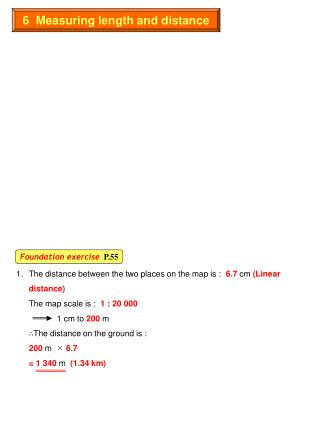DownloadDownload Presentation6 Measuring length and distance

# 6 Measuring length and distance

Télécharger la présentation## 6 Measuring length and distance

- - - - - - - - - - - - - - - - - - - - - - - - - - - E N D - - - - - - - - - - - - - - - - - - - - - - - - - - -
##### Presentation Transcript

1. 6 Measuring length and distance Foundation exerciseP.55 1. The distance between the two places on the map is : 6.7 cm (Linear distance)The map scale is : 1 : 20 0001 cm to 200 m∴The distance on the ground is :200 m  6.7 = 1 340 m (1.34 km)

2. 2. The length of Deep Bay Road on the map is 5 cm. The map scale is : 1 : 20 000 1 cm to 200 m ∴The length of Deep Bay Road on the ground is :200 m  5 = 1 000 m3. a) The length of the river on the map is 11.5 cm. The map scale is : 1 : 20 000 1 cm to 200 m ∴The length of the river on the ground is :200 m  11.5 = 2 300 mb) The distance on the map is : 10.8 cm (Linear distance) The map scale is : 1 : 20 000 1 cm to 200 m ∴The distance on the ground is :200 m  10.8 = 2 160 mc) With reference to 2b), the length of the river is 2 300 m. ∴The number of hours need to complete the journey is :2 300 m 5m = 460 minutes = 7.67 hours (i.e. 460 minutes  60 minutes)

3. 4. The longest section of the park on the map is 4.2 cm. (Refer to right diagram.) The map scale is : 1 : 20 000 1 cm to 200 m ∴The longest section of the park is :200 m  4.2 = 840 m5. a) The length of the light transit railway on the map is 5.5 cm The map scale is : 1 : 20 000 1 cm to 200 m ∴The length of the light transit railway on the ground is :200 m  5.5 = 1 100 mb) With reference to 5a), the length of the railway is 1 100 m. ∴The time needed to complete the journey is :1 100 m 60 km = 1 100 m 60 000 m = 0.0183 hour = 1.1 minutes (i.e. 60 minutes  0.0183)